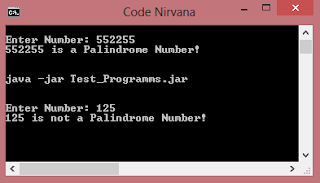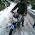# Java Program To Check Palindrome Number

### What is a Palindrome Number?

A palindromic number or palindrome number is a number that remains the same when its digits are reversed. Like 16461.

### Logic For Palindrome:

After understanding the meaning of palindrome number, its get easier for us to build a program to check whether a number is palindrome or not? So, as per the above definition we will take a number and than check if it remains same after getting reversed! so, the main logic behind this program is to take a number(input) and reverse it and than compare the reversed number with the number at the time of input. Lets see how to do it...
Source Code:
```/* Palindrome Program In JAVA
*/
import java.util.Scanner;
class Palindrome{
public static void main(String args[]){
System.out.print("Enter Number: ");
int n = num;
//reversing number
int rev=0,rmd;
while(num > 0)
{
rmd = num % 10;
rev = rev * 10 + rmd;
num = num / 10;
}
if(rev == n)
System.out.println(n+" is a Palindrome Number!");
else
System.out.println(n+" is not a Palindrome Number!");
}
}
```

Output:#### Tutorial: Palindrome Program In Javaexplain me in detail code for palindromeThe above article explains about palindrome and its code in detail!
What you want to know, or which part you didn't understand?sir , please explain 12 and 16 line in detail .thankyou in advanceWell before explaining I will suggest you to first run this program on paper (manually) for better understanding of the logic used!

Here, rev is for reverse and rmd for remainder.
In line 12 we created two variables rev and rmd of int type.
and in line 16 we are assigning values to variable rev in every run. here's the explanation:
rev = rev * 10 + rmd;
In the first run rmd = 2 and rev = 0*10 + 2 == 2
in second run rmd = 1 and rev = 2*10 + 1 == 21
and in the third run our condition becomes false and loop breaks!
Than the reverse of 12 is 21 , which is the required result....

Hope you got our point, for more help feel free to contact us!Thanks for the explanation! :)Our pleasure! Keep Visiting.....i cant understand same example plzzzz help mesir can you explain me the full logic of palindrome how it is done?Palindrome is just a number which remains the same on reversing it! So the logic is same as Reverse Number and further we just compared the reversed number with the input!
Hope this helps to get the logic behind this!sir, pls explain form 10 to 19 linepalindrome is means to check it can reverse, so we getting number after dat we createing input after i cant understand.. pls help me wat logic s going inside it to check palinderome or nor pls help meFirst input number, make a copy of that num variable to variable n, apply reverse number logic to n and store reversed number in variable rev, finally check whether rev == num.... if condition is true means palindrome otherwise not!
Hope this gives you better understanding about the logic and program concept!Number reversing process is done there! nothing much to explain... check reverse number post @ our blog.Thanks sir, i understand ... thanks vry much for instead if scanner why we cannot use DataInputStream obj= new DataInputStream(System.in);hi satish

BufferedReader, is a character stream I/O class. Character streams provide a convenient way for input and output in terms of characters (Unicode). BufferedReader is mostly used for taking input from the console, System.in. It takes an InputStreamReader object as an argument.

A Scanner can do all that a BufferedReader or a DataInputStream can do and at the same level of efficiency as well. However, in addition a Scanner can parse the underlying stream for primitive types and strings using regular expressions. It can also tokenize the underlying stream with the delimiter of your choice. It can also do forward scanning of the underlying stream disregarding the delimiter.Sir,
How do i check weather a String is palindrome or not?@ZeeShan Checking string palindrome is very easy using StringBuilder!
check the below function for checking whether string is palindrome of not:
public static boolean isPalindrome(String S) {
String rev = new StringBuilder(S).reverse().toString();
if( S.equals( rev ) ) return true;
return false;
}Following comparison is not happening when a palindrome number is given e.g. 454

if(rev == n)
System.out.println(n+" is a Palindrome Number!");

reversed number is not equaling to the number inputted ..please let us know@Srinath I tested the above code again and its working fine!
check here: ideone.com/H7WSmwcan u tell me how in d second run rev=1???help sir to check twin primes ...........{twin primes[3,5], [5,7], [11,13]....}This comment has been removed by the author.hi sir

i was wondering if you could help me solve this problem?

Zoe likes palindromes, and thinks them to be nice. A palindrome is just an integer that reads the same backwards and forwards - so 6, 11 and 121 are all palindromes, while 10, 12, 223 and 2244 are not (even though 010=10, we don't consider leading zeroes when determining whether a number is a palindrome).

She recently became interested in squares as well, and formed the definition of a nice and square number - it is a number that is a palindrome and the square of a palindrome at the same time. For instance, 1, 9 and 121 are nice and square (being palindromes and squares, respectively, of 1, 3 and 11), while 16, 22 and 676 are not nice and square: 16 is not a palindrome, 22 is not a square, and while 676 is a palindrome and a square number, it is the square of 26, which is not a palindrome.

Now he wants to search for bigger nice and square numbers. Your task is, given an interval Zoe is searching through, to tell her how many nice and square numbers are there in the interval, so she knows when she has found them all.

Input
The first line of the input gives the number of test cases, T. T lines follow. Each line contains two integers, A and B - the endpoints of the interval Zoe is looking at.

Output
For each test case, output one line containing "Case #x: y", where x is the case number (starting from 1) and y is the number of fair and square numbers greater than or equal to A and smaller than or equal to B.

Limits

Small dataset
1 ≤ T ≤ 100. 1 ≤ A ≤ B ≤ 1000.

Large dataset
1 ≤ T ≤ 10000. 1 ≤ A ≤ B ≤ 1014.

Sample

Input
3
1 4
10 120
100 1000

Output
Case #1: 2
Case #2: 0
Case #3: 2This problem is from Google Code Jam 2013 Qualification round.
Many posts/editorials already available on this, you can check there!if taking 121 as input
while loop will continue till it finds num<=0
in last and thirds time logic will store num=1 which further makes rev = 12+10+1;
rev=n; satisfies condition hence it is palindrome.if rev=0 so how is rev=rev*10+rmd?It will be '0*10+rmd' in first run, means the last digit(rmd) will be stored in rev first!
Tryout this logic manually and you will get my point.

Feel free to ask for any help!Very Clear! Thank you so muchIf a wrote my program in my way as in
Class palindrome
{
void main(int n)
{
Int r,rev=0,d=n;
while(d>0)
{
r=d%10;
rev=rev*10+r;
d=d/10;
}
if(d==n)
System.out.println("palindrome");
else
System.out.println("not a palindrome number");
}
}

M trying to make this program run on my computer bt d output for d program is not correct as per my inputted value... Can u help me find out my mistake....If a wrote my program in my way as in
Class palindrome
{
void main(int n)
{
Int r,rev=0,d=n;
while(d>0)
{
r=d%10;
rev=rev*10+r;
d=d/10;
}
if(d==n)
System.out.println("palindrome");
else
System.out.println("not a palindrome number");
}
}

M trying to make this program run on my computer bt d output for d program is not correct as per my inputted value... Can u help me find out my mistake....I can't understand logic which is used in this program. Can u briefly explain in it...Sir why num= num/10 is wrritten what its use